# Venture Capital Valuation Method

The venture capital method (VC) in private equity investing is a method to value the investment in an existing start-up company. The method starts from the expected exit value, which we discount to today. That value, called the post-money value (POST), is crucial to valuing the company.

On this page, we discuss the venture capital valuation model, go over a problem set to get a thorough understanding of the approach, and finally implement the approach to using a venture capital valuation model template. The spreadsheet can be downloaded below.

## Venture capital valuation model

Let’s start by discussing the possible cases we can encounter when using the VC valuation method. There’s two basic versions, the single round method and the multiple rounds method. As the names suggest, the kind of method depends on the number of investment rounds we are expecting. Once we know whether or not we will have a single round or multiple rounds, we can calculate a number of metrics.

In particular, we need to calculate the pre-money value (PRE)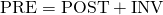Once we have that, we can calculate the VC ownership. There are two methods we can use, the NPV method or the IRR method. In the case of the NPV method, we do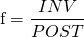When using the IRR method, we apply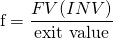Both methods will yield the same result. f is sometimes also called the fractional ownership required. Next, we can calculate the number of shares that have to be issued. Often, we know the number of shares the founders want to keep. The number of shares to be issued is then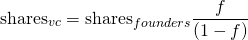Finally, the price per share equals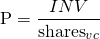In the case of multiple rounds, the same logic applies. In that case, we start from the exit value at the very end. Then we work our way back. Both the multiple rounds and the single round approach are illustrated in the spreadsheet below.

Venture capital method valuation example

Let’s turn to an example. The following table implements the steps of a single round venture capital model xls: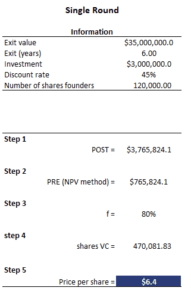As for a venture capital method problem set with multiple rounds, the following table implements such a case: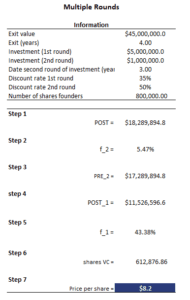Summary

We discussed the venture capital method. It is an important approach used by analysts to value an investment in a start-up or tech company. The method is easy to use and requires just a few formulae.